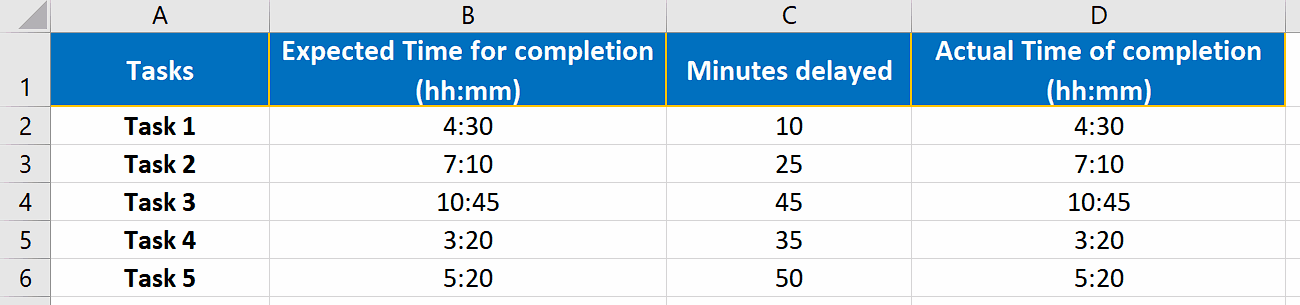# How to add minutes to time in Excel

In this tutorial we’ll learn how to add minutes to a time value. This is a tricky task because you need to format the minutes properly so that Excel recognizes the numbers as minutes and only then you can add minutes to the time value.

## Example 1 The problem of adding simple numbers to time

Before learning how to add numbers to the time specific value, we’ll try to understand the problem associated with adding simple numbers to time values.

We can see that the delayed minutes did not get added to the completion time and column D has the same values as column B. To resolve this issue let’s follow the steps mentioned below.It may seem to be a very simple idea to add some minutes to a time value in Excel, however, it is not as simple as it may seem. When you are keeping track of the time as a project manager or duty hours measurement is your responsibility then you will definitely face this situation when you need to add minutes or sometimes add hours to the time data.

### Step 1 – Format Minutes delayed to reflect time values– To resolve the aforementioned problem, we’ll have to change the format i.e., rewrite the numbers in proper format i.e., hh:mm (00:10) manually. Then Excel can recognize the numbers as time values.

– When we change the format to hh:mm the results will start updating automatically because of the additional formula implemented to calculate the actual completion time of the tasks as shown above.

So this is how we can resolve the dilemma of adding minutes to time values by changing the formats of the number to proper time format.